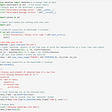# AI for Trading Series №3: Regression

This series focuses on two main points:

• Checking and transforming data.
• Regression.

There are some visual ways to check if a distribution is normally distributed or not:

• Box-whisker plots helps us visually check if a distribution is symmetric or skewed.
• A histogram lets us check if a distribution is symmetric/skewed, and if it has fat tails.
• QQ plots help us compare any two distributions, so they can be used to compare distributions other than the normal distribution.

There are three hypothesis tests that can be used to decide if a data distribution is normal:

• Shapiro-Wilk test
• D’Agostino-Pearson test
• Kolmogorov-Smirnov test.

Each of these produce p-value, and if the p-value is small enough, say 0.05 or less, we can say with a 95% confidence that the data is not normally distributed.

Shapiro-Wilk tends to perform better in a broader set of cases compared to the D’Agostino-Pearson test. In part, this is because the D’Agostino-Pearson test is used to look for skewness and kurtosis that do not match a normal distribution, so there are some odd non-normal distributions for which it doesn’t detect non-normality, where the Sharpiro-Wilk would give the correct answer.

The Kolmogorov Smirnov test can be used to compare distributions other than the normal distribution, so it’s similar to the QQ plot in its generality. To do a normality test, we would first rescale the data distribution (subtract the mean and divide by its standard deviation), then compare the rescaled data distribution with the standard normal distribution (which has a mean of zero and standard deviation of 1). In general, the Shapiro-WIlk test tends to be a better test than the Kolmogorov Smirnov test, but not in all cases.

So in summary, if you want to be thorough, you can use all three tests. If you only want to use one test, use the Shapiro-Wilk test. For a sanity check, visualize your data distribution with a histogram, box-whisker plot, and/or a QQ plot.

Corresponding python implementation of Normality can be found here

# Heteroskedasticity

One of the assumptions of linear regression is that its input data are homoscedastic. A visual way to check if the our data is homoscedastic is a scatter plot.

If our data is heteroscedastic, a linear regression estimate of the coefficients may be less accurate (further from the actual value), and we may get a smaller p-value than should be expected, which means we may assume (incorrectly) that we have an accurate estimate of the regression coefficient, and assume that it’s statistically significant when it’s not.

# Breusch-Pagan Test

The Breusch-Pagan (BP) test is one of the most common tests for heteroskedasticity. It begins by allowing the heteroskedasticity process to be a function of one or more of your independent variables, and it’s usually applied by assuming that heteroskedasticity may be a linear function of all the independent variables in the model.

# Transforming Data

In order to transform our data into a normal data, we can feed our data into log function.

In order to make data homoscedastic, we usually take the rate of return from one day to the next and then pass that data through log function.

# Box-cox Transformation

Box-cox transformation is used to make our data `normal + homoscedastic`. A monotonic transformation changes the values of dataset but preserves their relative order. Box-cox dataset takes in dataset and outputs a more normally distributed dataset.

# Linear Regression

Taking an example of purchasing the house. Here, we can say that the price of the house depends upon area. For this, we will find area covered by each house as well as their price. We find a coefficient to multiply by the area and add constant term (intercept). This is equation of a straight line. Upon plotting this straight line we want it to pass through almost all the data points.

We can measure how well the line fits the data by measuring the vertical difference between the points and the line. An optimal line is the one that reduces these differences. The process of finding the optimal regression line is called ordinary least squares. After plotting the optimal line, there might be some outliers. The difference between best fit line at each point is called Residual (error terms).

# Evaluating Model

We can evaluate our model to check if its the best fit by the following methods

1. R-squared
3. F-test

# Multi-variate Regression

If we chose more than one depended variable that we wish to predict. For example, we try to predict not just housing price but also home’s electricity consumption and gas consumption. This is known as multi-variate regression. When we are using more than one in-depended variable to predict more than one depended variable, it is called multi-variate multiple regression.

The corresponding python implementation using sklearn’s `LinearRegression` can be found here

## More from Purva Singh

Hi! I am a tech enthusiast currently working on leveraging language technologies to solve financial use-cases! View my work here: https://purvasingh96.github.io

Love podcasts or audiobooks? Learn on the go with our new app.

## Endogeneity And Why You Should Keep It In Mind as a Data Scientist.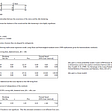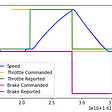## Web Scraping Hertz Car Inventory with Python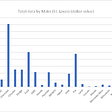## Winning the Kaggle Google Brain — Ventilator Pressure Prediction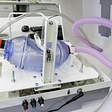## 5 “Must Know” Technical Indicators for building an Automated Trading System## Why am I writing a book on ‘Data and AI’?## Arithmetic Operation Inside the String with Python eval() Function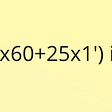## GRIB Outputs and the Race to Mackinac## Purva Singh

Hi! I am a tech enthusiast currently working on leveraging language technologies to solve financial use-cases! View my work here: https://purvasingh96.github.io

## Liquor Sales Prediction## Airbnb as a Metric to Assess Health of Major US Cities, Price Prediction, and Text Analysis of…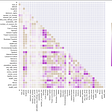## Detecting Room Occupancy Using Machine Learning and Sensor Data## Simple way to visualize financial data. MplFinance